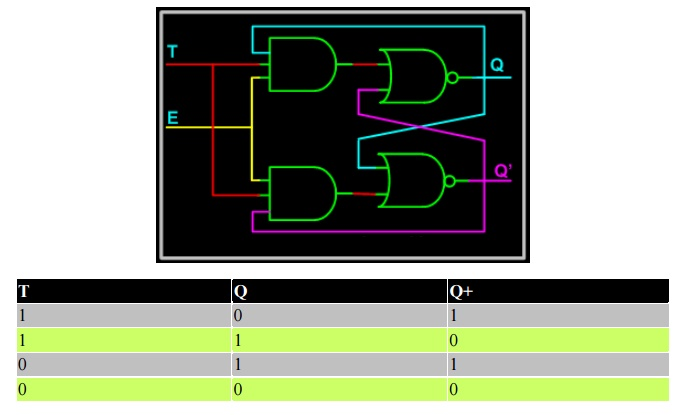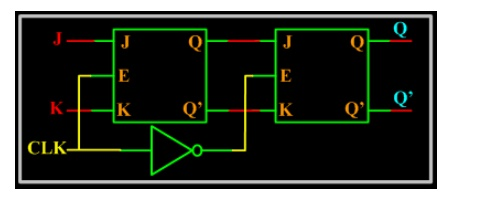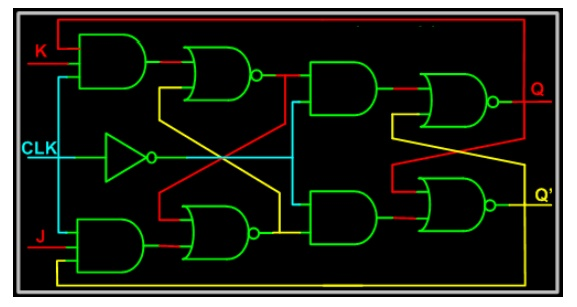Home | | Digital Electronics | Latches and Flip-Flops

# Latches and Flip-Flops

There are two types of sequential circuits. · Synchronous Circuits. · Asynchronous Circuits.

LATCHES AND FLIP-FLOPS

There are two types of sequential circuits.

·              Synchronous Circuits.

·              Asynchronous Circuits.

As seen in last section, Latches and Flip-flops are one and the same with a slight variation: Latches have level sensitive control signal input and Flip-flops have edge sensitive control signal input. Flip-flops and latches which use this control signals are called synchronous circuits. So if they don't use clock inputs, then they are called asynchronous circuits.

## 1. RS Latch

RS latch have two inputs, S and R. S is called set and R is called reset. The S input is used to produce HIGH on Q ( i.e. store binary 1 in flip-flop). The R input is used to produce LOW on Q (i.e. store binary 0 in flip-flop). Q' is Q complementary output, so it always holds the opposite value of Q. The output of the S-R latch depends on current as well as previous inputs or state, and its state (value stored) can change as soon as its inputs change. The circuit and the truth table of RS latch is shown below.The operation has to be analyzed with the 4 inputs combinations together with the 2 possible previous states.

·              When S = 0 and R = 0: If we assume Q = 1 and Q' = 0 as initial condition, then output Q after input is applied would be Q = (R + Q')' = 1 and Q' = (S + Q)' = 0. Assuming Q =             0 and Q' = 1 as initial condition, then output Q after the input applied would be Q = (R + Q')' = 0 and Q' = (S + Q)' = 1. So it is clear that when both S and R inputs are LOW, the output is retained as before the application of inputs. (i.e. there is no state change).

When S = 1 and R = 0: If we assume Q = 1 and Q' = 0 as initial condition, then output Q after input is applied would be Q = (R + Q')' = 1 and Q' = (S + Q)' = 0. Assuming Q = 0 and Q' = 1 as initial condition, then output Q after the input applied would be Q = (R + Q')' = 1 and Q' = (S + Q)' = 0. So in simple words when S is HIGH and R is LOW, output Q is HIGH.

When S = 0 and R = 1: If we assume Q = 1 and Q' = 0 as initial condition, then output Q after input is applied would be Q = (R + Q')' = 0 and Q' = (S + Q)' = 1. Assuming Q = 0 and Q' = 1 as initial condition, then output Q after the input applied would be Q = (R + Q')' = 0 and Q' = (S + Q)' = 1. So in simple words when S is LOW and R is HIGH, output Q is LOW.

·              When S = 1 and R =1 : No matter what state Q and Q' are in, application of 1 at input of NOR gate always results in 0 at output of NOR gate, which results in both Q and Q' set to LOW (i.e. Q = Q'). LOW in both the outputs basically is wrong, so this case is invalid.

It is possible to construct the RS latch using NAND gates (of course as seen in Logic gates section). The only difference is that NAND is NOR gate dual form (Did I say that in

Logic gates section?). So in this case the R = 0 and S = 0 case becomes the invalid case. The circuit and Truth table of RS latch using NAND is shown below.If you look closely, there is no control signal (i.e. no clock and no enable), so this kind of latches or flip-flops are called asynchronous logic elements. Since all the sequential circuits are built around the RS latch, we will concentrate on synchronous circuits and not on asynchronous circuits.

## 2. RS Latch with Clock

We have seen this circuit earlier with two possible input configurations: one with level sensitive input and one with edge sensitive input. The circuit below shows the level sensitive RS latch. Control signal "Enable" E is used to gate the input S and R to the RS Latch. When Enable E is HIGH, both the AND gates act as buffers and thus R and S appears at the RS latch input and it functions like a normal RS latch. When Enable E is LOW, it drives LOW to both inputs of RS latch. As we saw in previous page, when both inputs of a NOR latch are low, values are retained (i.e. the output does not change).2.1 Set up and Hold time

For synchronous flip-flops, we have special requirements for the inputs with respect to clock signal input. They are

·              Setup Time: Minimum time period during which data must be stable before the clock makes a valid transition. For example, for a posedge triggered flip-flop, with a setup time of 2 ns, Input Data (i.e. R and S in the case of RS flip-flop) should be stable for at least 2 ns before clock makes transition from 0 to 1.

·              Hold Time: Minimum time period during which data must be stable after the clock has made a valid transition. For example, for a posedge triggered flip-flop, with a hold time of 1 ns. Input Data (i.e. R and S in the case of RS flip-flop) should be stable for at least 1 ns after clock has made transition from 0 to 1.

If data makes transition within this setup window and before the hold window, then the flip-flop output is not predictable, and flip-flop enters what is known as meta stable state. In this state flip-flop output oscillates between 0 and 1. It takes some time for the flip-flop to settle down. The whole process is called metastability. You could refer to tidbits section to know more information on this topic.

The waveform below shows input S (R is not shown), and CLK and output Q (Q' is not shown) for a SR posedge flip-flop.## 3. D Latch

The RS latch seen earlier contains ambiguous state; to eliminate this condition we can ensure that S and R are never equal. This is done by connecting S and R together with an inverter. Thus we have D Latch: the same as the RS latch, with the only difference that there is only one input, instead of two (R and S). This input is called D or Data input. D latch is called D transparent latch for the reasons explained earlier. Delay flip-flop or delay latch is another name used. Below is the truth table and circuit of D latch.

In real world designs (ASIC/FPGA Designs) only D latches/Flip-Flops are used.Below is the D latch waveform, which is similar to the RS latch one, but with R removed.## 4. JK Latch

The ambiguous state output in the RS latch was eliminated in the D latch by joining the inputs with an inverter. But the D latch has a single input. JK latch is similar to RS latch in that it has 2 inputs J and K as shown figure below. The ambiguous state has been eliminated here: when both inputs are high, output toggles. The only difference we see here is output feedback to inputs, which is not there in the RS latch.## 5. T Latch

When the two inputs of JK latch are shorted, a T Latch is formed. It is called T latch as, when input is held HIGH, output toggles.## 6. JK Master Slave Flip-Flop

All sequential circuits that we have seen in the last few pages have a problem (All level sensitive sequential circuits have this problem). Before the enable input changes state from HIGH to LOW (assuming HIGH is ON and LOW is OFF state), if inputs changes, then another state transition occurs for the same enable pulse. This sort of multiple transition problem is called racing.

If we make the sequential element sensitive to edges, instead of levels, we can overcome this problem, as input is evaluated only during enable/clock edges.In the figure above there are two latches, the first latch on the left is called master latch and the one on the right is called slave latch. Master latch is positively clocked and slave latch is negatively clocked.Study Material, Lecturing Notes, Assignment, Reference, Wiki description explanation, brief detail
Digital Electronics : Synchronous and Asynchronous Sequential Circuits : Latches and Flip-Flops |